# RD Sharma Solutions - Chapter 14 - Compound Interest (Part - 2), Class 8, Maths Notes | Study RD Sharma Solutions for Class 8 Mathematics - Class 8

## Class 8: RD Sharma Solutions - Chapter 14 - Compound Interest (Part - 2), Class 8, Maths Notes | Study RD Sharma Solutions for Class 8 Mathematics - Class 8

The document RD Sharma Solutions - Chapter 14 - Compound Interest (Part - 2), Class 8, Maths Notes | Study RD Sharma Solutions for Class 8 Mathematics - Class 8 is a part of the Class 8 Course RD Sharma Solutions for Class 8 Mathematics.
All you need of Class 8 at this link: Class 8

PAGE NO 14.14:

Question 1:

Compute the amount and the compound interest in each of the following by using the formulae when:
(i) Principal = Rs 3000, Rate = 5%, Time = 2 years
(ii) Principal = Rs 3000, Rate = 18%, Time = 2 years
(iii) Principal = Rs 5000, Rate = 10 paise per rupee per annum, Time = 2 years
(iv) Principal = Rs 2000, Rate = 4 paise per rupee per annum, Time = 3 years
(v) Principal = Rs 12800, Rate =  7(1/2)%, Time = 3 years
(vi) Principal = Rs 10000, Rate 20% per annum compounded half-yearly, Time = 2 years
(vii) Principal = Rs 160000, Rate = 10 paise per rupee per annum compounded half-yearly, Time = 2 years.

Applying the rule A = P(1 + R/100)n on the given situations, we get:

(i)A = 3,000(1 + 5/100)²

= 3,000(1.05)²

= Rs 3,307.50

Now,CI = A − P

= Rs 3,307.50 − Rs 3,000

= Rs 307.50

(ii)A = 3,000(1 + 18/100)²

= 3,000(1.18)²

= Rs 4,177.20

Now,CI = A − P

= Rs 4,177.20 − Rs 3,000

= Rs 1,177.20

(iii)A = 5,000(1 + 10/100)²

= 5,000(1.10)²

= Rs 6,050

Now,CI = A − P

= Rs 6,050 − Rs 5,000

= Rs 1,050

(iv)A = 2,000(1 + 4/100)³

= 2,000(1.04)³

= Rs 2,249.68

Now,CI = A − P

= Rs 2,249.68 − Rs 2,000

= Rs 249.68

(v)A = 12,800(1 + 7.5/100)³

= 12,800(1.075)³

= Rs 15,901.40

Now,CI = A − P

= Rs 15,901.40 − Rs 12,800

= Rs 3,101.40

(vi)A = 10,000(1 + 20/200)4

= 10,000(1.1)

= Rs 14,641

Now,CI = A − P

= Rs 14,641 − Rs 10,000

= Rs 4,641

(vii)A = 16,000(1 + 10/200)4

= 16,000(1.05)4

= Rs 19,448.1

Now,CI = A − P

= Rs 19,448.1 − Rs 16,000

= Rs 3,448.1

PAGE NO 14.14:

Question 2:

Find the amount of Rs 2400 after 3 years, when the interest is compounded annually at the rate of 20% per annum.

Given: P = Rs 2,400

R = 20% p.a.

n = 3 years

We know that amount A at the end of n years at the rate R% per annum when the interest is compounded annually is given by

A = P(1 + R/100)n

∴ A = 2,400(1 + 20100)³

= 2,400(1.2)³

= 4,147.20

Thus, the required amount is Rs 4,147.20.

PAGE NO 14.14:

Question 3:

Rahman lent Rs 16000 to Rasheed at the rate of 12.5% per annum compound interest. Find the amount payable by Rasheed to Rahman after 3 years.

Given: P = Rs 16,000

R = 12.5% p.a.

n = 3 years

We know that amount A at the end of n years at the rate R% per annum when the interest is compounded annually is given by

A = P(1 + R/100)n.

∴ A = 16,000(1 + 12.5/100)³

= 16,000(1.125)³

= 22,781.25

Thus, the required amount is Rs 22,781.25.

PAGE NO 14.14:

Question 4:

Meera borrowed a sum of Rs 1000 from Sita for two years. If the rate of interest is 10% compounded annually, find the amount that Meera has to pay back.

Given: P = Rs 1,000

R = 10% p.a.

n = 2 years

We know that amount A at the end of n years at the rate R% per annum when the interest is compounded annually is given by

A = P(1 + R/100).

∴ A = 1,000(1 + 10/100)²

= 1,000(1.1)² = 1,210

Thus, the required amount is Rs 1,210.

PAGE NO 14.14:

Question 5:

Find the difference between the compound interest and simple interest. On a sum of Rs 50,000 at 10% per annum for 2 years.

Given: P = Rs 50,000

R = 10% p.a.

n = 2 years

We know that amount A at the end of n years at the rate R% per annum when the interest is compounded annually is given by

A = P(1 + R/100)

.∴ A = Rs 50,000(1 + 10/100)²

= Rs 50,000(1.1)²

= Rs 60,500

Also,CI = A − P

= Rs 60,500 −  Rs 50,000

= Rs 10,500

We know that: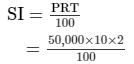∴ Difference between CI and SI = Rs 10,500 − Rs 10,000

= Rs 500

PAGE NO 14.15:

Question 6:

Amit borrowed Rs 16000 at 17.5% per annum simple interest. On the same day, he lent it to Ashu at the same rate but compounded annually. What does he gain at the end of 2 years?

Amount to be paid by Amit: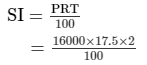= Rs 5,600

Amount gained by Amit:A = P(1 + R/100)n

= Rs 16,000(1 + 17.5/100)²

= Rs 16,000(1.175)²

= Rs 22,090

We know that:CI = A − P

= Rs 22,090 − Rs 16,000     = Rs 6090

Amit's gain in the whole transaction = Rs 6,090 − Rs 5,600

= Rs 490

PAGE NO 14.15:

Question 7:

Find the amount of Rs 4096 for 18 months at 12.5% per annum, the interest being compounded semi-annually.

Given:P = Rs 4,096

R = 12.5% p.a.

n = 18 months = 1.5 years

We have:A = P(1 + R/100)n

When the interest is compounded semi − annually, we have:

A = P(1 + R/200)²n

= Rs 4,096(1 + 12.5/200)³

= Rs 4,096(1.0625)³

= Rs 4,913

Thus, the required amount is Rs 4,913.

PAGE NO 14.15:

Question 8:

Find the amount and the compound interest on Rs 8000 for 1.5 years at 10% per annum, compounded half-yearly.

Given: P = Rs 8,000

R = 10% p.a.

n = 1.5 years

When compounded half − yearly, we have:

A = P(1 + R/200)²n

= Rs 8,000(1 + 10/200)³

= Rs 8,000(1.05)³

= Rs 9,261

Also,CI = A − P

= Rs 9,261 − Rs 8,000

= Rs 1,261

PAGE NO 14.15:

Question 9:

Kamal borrowed Rs 57600 from LIC against her policy at 12.5% per annum to build a house. Find the amount that she pays to the LIC after 1.5 years if the interest is calculated half-yearly.

Given: P = Rs 57,600

R = 12.5% p.a.

n = 1.5 years

When the interest is compounded half − yearly, we have:

A = P(1 + R/200)²n

= Rs 57,600(1 + 12.5/200)³

= Rs 57,600(1.0625)³

= Rs 69,089.06

Thus, the required amount is Rs 69,089.06.

PAGE NO 14.15:

Question 10:

Abha purchased a house from Avas Parishad on credit. If the cost of the house is Rs 64000 and the rate of interest is 5% per annum compounded half-yearly, find the interest paid by Abha after one year and a half.

Given:P = Rs 64,000

R = 5% p.a.

n = 1.5 years

When the interest is compounded half − yearly, we have:

A = P(1 + R/200)²n

= Rs 64,000(1 + 5/200)³

= Rs 64,000(1.025)³

= Rs 68,921

Also,CI = A − P

= Rs 68,921 − Rs 64,000

= Rs 4,921

Thus, the required interest is Rs 4,921.

PAGE NO 14.15:

Question 11:

Rakesh lent out Rs 10000 for 2 years at 20% per annum, compounded annually. How much more he could earn if the interest be compounded half-yearly?

Given:P = Rs 10,000

R = 20% p.a.

n = 2 years

A = P(1 + R/100)n

=  Rs 10,000(1 + 20/100)²

= Rs 10,000(1.2)²

= Rs 14,400

When the interest is compounded half − yearly, we have:A = P(1 + R/200)²n

= Rs 10,000(1 + 20/200)

= Rs 10,000(1.1)4

= Rs 14,641

Difference = Rs 14,641 − Rs 14,400

= Rs 241

PAGE NO 14.15:

Question 12:

Romesh borrowed a sum of Rs 245760 at 12.5% per annum, compounded annually. On the same day, he lent out his money to Ramu at the same rate of interest, but compounded semi-annually. Find his gain after 2 years.

Given: P = Rs 245,760

R = 12.5% p.a.

n = 2 years

When compounded annually, we have:A = P(1 + R/100)n

= Rs 245,760(1 + 12.5/100)²

= Rs 311,040

When compounded semi − annually,

we have:A = P(1 + R/200)²n

= Rs 245,760(1 + 12.5/200)4

= Rs 245,760(1.0625)

= Rs 313,203.75

Romesh's gain = Rs 313,203.75 − Rs 311,040

= Rs 2,163.75

PAGE NO 14.15:

Question 13:

Find the amount that David would receive if he invests Rs 8192 for 18 months at 12.5% per annum, the interest being compounded half-yearly.

Given:P = Rs 8,192

R = 12.5% p.a.

n = 1.5 years

When the interest is compounded half − yearly, we have:A = P(1 + R/200)²n

= Rs 8,192(1 + 12.5/200)³

= Rs 8,192(1.0625)³

= Rs 9,826

Thus, the required amount is Rs 9,826.

PAGE NO 14.15:

Question 14:

Find the compound interest on Rs 15625 for 9 months, at 16% per annum, compounded quarterly.

Given: P = Rs 15,625

R = 16% = 164 = 4% quarterly

n = 9 months = 3 quarters

We know that:A = P(1 + R/100)n

= Rs 15,625(1 + 4/100)³

= Rs 15,625(1.04)³

= Rs 17,576

Also,CI = A − P

= Rs 17,576 − Rs 15,625

= Rs 1,951

Thus, the required compound interest is Rs 1,951.

PAGE NO 14.15:

Question 15:

Rekha deposited Rs 16000 in a foreign bank which pays interest at the rate of 20% per annum compounded quarterly, find the interest received by Rekha after one year.

Given:P = Rs 16,000

R = 20% p.a.

n = 1 year

We know that:A = P(1 + R/100)n

When compounded quarterly, we have:A = P(1 + R/400)4n

= Rs 16,000(1 + 20/400)4

= Rs 16,000(1.05)4

= Rs 19,448.10

Also,CI = A − P

= Rs 19,448.1 − Rs 16,000

= Rs 3,448.10

Thus, the interest received by Rekha after one year is Rs 3,448.10.

PAGE NO 14.15:

Question 16:

Find the amount of Rs 12500 for 2 years compounded annually, the rate of interest being 15% for the first year and 16% for the second year.

Given: P = Rs 12,500

R = 15% p.a.

R2 = 16% p.a.

∴ Amount after two years = P(1 + R1/100)(1 + R2/100)

= Rs 12,500(1 + 15/100)(1 + 16/100)

= Rs 12,500(1.15)(1.16)

= Rs 16,675

Thus, the required amount is Rs 16,675.

PAGE NO 14.15:

Question 17:

Ramu borrowed Rs 15625 from a finance company to buy a scooter. If the rate of interest be 16% per annum compounded annually, what payment will he have to make after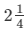years?

Given: P = Rs 15,625

R = 16% p.a.

n =years

∴ Amount afteryears = P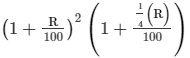= Rs 15,625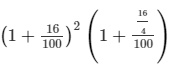= Rs 15,625(1.16)²(1.04)

= Rs 21,866

Thus, the required amount is Rs 21,866.

PAGE NO 14.15:

Question 18:

What will Rs 125000 amount to at the rate of 6%, if the interest is calculated after every 3 months?

Because interest is calculated after every 3 months, it is compounded quarterly.

Given:

P = Rs 125,000

R = 6% p.a. = 6/4% quarterly = 1.5% quarterly

n = 4

So,A = P(1 + R/100)

= 125,000(1 + 1.5/100)4

= 125,000(1.015)4

= 132,670 (approx)

Thus, the required amount is Rs 132,670.

PAGE NO 14.15:

Question 19:

Find the compound interest at the rate of 5% for three years on that principal which in three years at the rate of 5% per annum gives Rs 12000 as simple interest.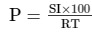According to the given values, we have: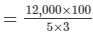= 80,000

The principal is to be compounded annually.

So, A = P(1 + R/100)n

= 80,000(1 + 5/100)³

= 80,000(1.05)³

= 92,610

Now,CI = A − P

= 92,610 − 80,000

= 12,610

Thus, the required compound interest is Rs 12,610.

PAGE NO 14.15:

Question 20:

A sum of money was lent for 2 years at 20% compounded annually. If the interest is payable half-yearly instead of yearly, then the interest is Rs 482 more. Find the sum.

A = P(1 + R/100)n

Also, P = A − CI

Let the sum of money be Rs x.

If the interest is compounded annually, then:

A1 = x(1 + 20/100)²

= 1.44x

∴ CI = 1.44x − x

= 0.44x         ...(1)

If the interest is compounded half − yearly, then:

A = x(1 + 10/100)4

= 1.4641x

∴ CI = 1.4641x − x

= 0.4641x    ...(2)

It is given that if interest is compounded half − yearly, then it will be Rs 482 more.

∴0.4641x = 0.44x + 482    [From (1) and (2)]

0.4641x − 0.44x = 482

0.0241x = 482

x = 482/0.0241

= 20,000

Thus, the required sum is Rs 20,000.

PAGE NO 14.15:

Question 21:

Simple interest on a sum of money for 2 years at 6.5% per annum is Rs 5200. What will be the compound interest on the sum at the same rate for the same period?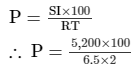= 40,000

Now,

A = P(1 + R/100)n

= 40,000(1 + 6.5/100)²

= 40,000(1.065)²

= 45,369

Also,CI = A − P

= 45,369 − 40,000

= 5,369

Thus, the required compound interest is Rs 5,369

PAGE NO 14.15:

Question 22:

Find the compound interest at the rate of 5% per annum for 3 years on that principal which in 3 years at the rate of 5% per annum gives Rs 1200 as simple interest.

We know that: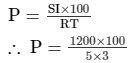= 8,000

Now,

A = P(1 + R/100)n

= 8,000(1 + 5/100)³

= 8,000(1.05)³    = 9,261

Now,CI = A − P

= 9,261 − 8,000

= 1,261

Thus, the required compound interest is Rs 1,261.

The document RD Sharma Solutions - Chapter 14 - Compound Interest (Part - 2), Class 8, Maths Notes | Study RD Sharma Solutions for Class 8 Mathematics - Class 8 is a part of the Class 8 Course RD Sharma Solutions for Class 8 Mathematics.
All you need of Class 8 at this link: Class 8Use Code STAYHOME200 and get INR 200 additional OFF

## RD Sharma Solutions for Class 8 Mathematics

88 docs

Track your progress, build streaks, highlight & save important lessons and more!

,

,

,

,

,

,

,

,

,

,

,

,

,

,

,

,

,

,

,

,

,

,

,

,

,

,

,

;